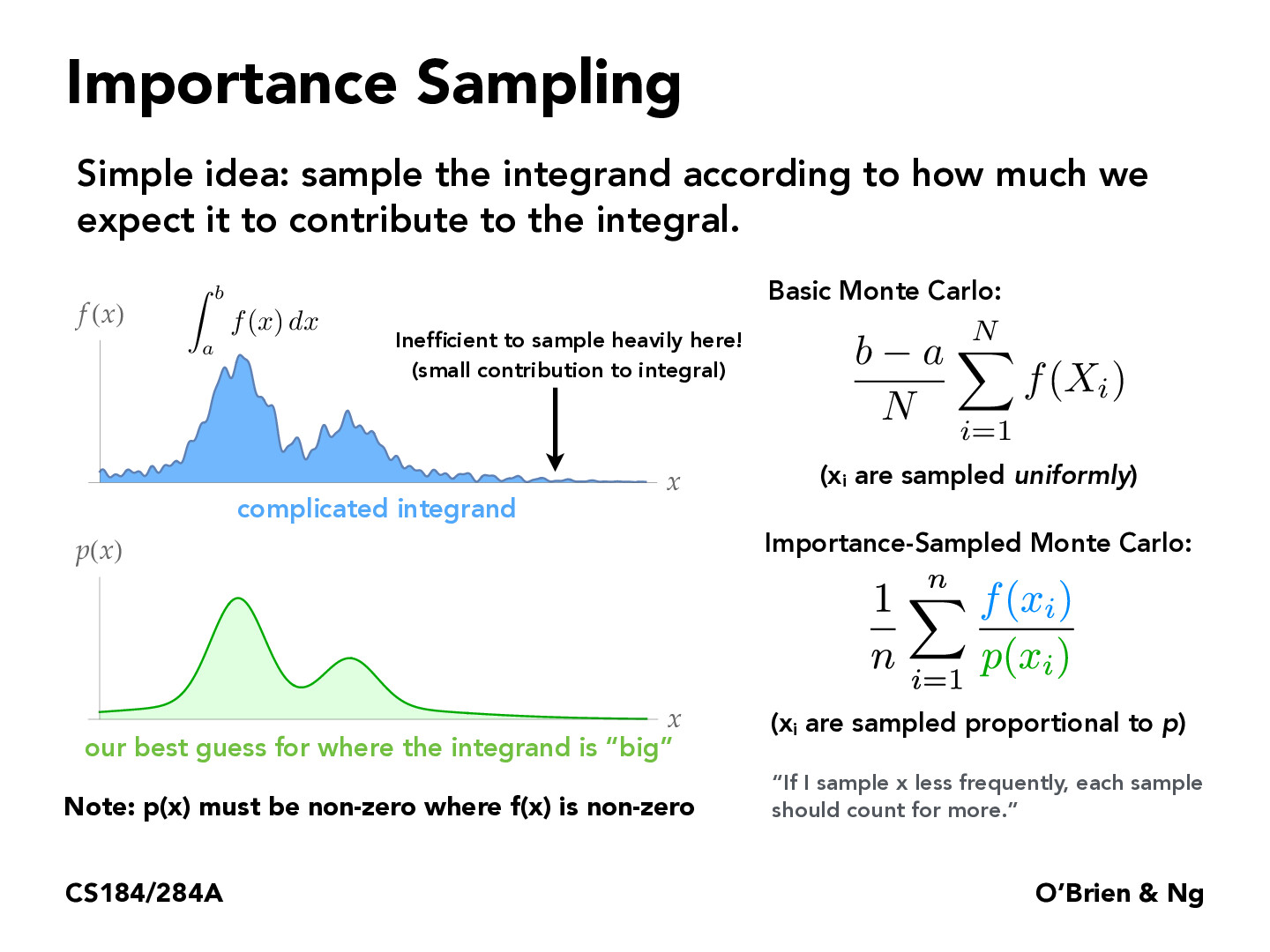Lecture 12: Monte Carlo Integration (40)yzliu567

For better understanding, we can consider the extreme case: $f(x) = C p(x)$. Then the sampling method is perfect because the variance is 0, and the integral is $C$.waleedlatif1

In using importance sampling, I understand that we want the underlying distribution to be similar to the 'actual' distribution, and we want to use pi that reflect those values to converge as soon as possible, but I was just curious what convergence means in this case and how to decide what underlying pdf is good enough for us to consider the integrand 'converged.'kkoujah

@waleedlatif1 In importance sampling, convergence refers to the accuracy and stability of the estimated integral as we use more and more samples from the importance distribution. The convergence rate depends on the choice of importance distribution and properties of the integrand. To decide if the integrand has converged, we can monitor the variance of the estimated integral over multiple runs with different numbers of samples. A common approach is to choose the importance distribution that has the highest overlap with the actual distribution or one that has a similar shape to the integrand.AlexSchedel

I am a little confused here as to why we want to wait the more out of the way samples more heavily. It would seem to me that a sample far from the actual mean should not be weighted because it will already drag us away from the mean of this function. Is the reason that if we sample the points without weighting we will get a smoother curve (as per the CLT?) or is there some other reason we would want to wait things?stexus

I had the same thoughts as Alex. If we're sampling the integrand according to how much we expect it to contribute to the full integral, why are we effectively punishing integrands that are more likely to be drawn and therefore more likely to contribute to the actual integral?jonathanlu31

I think the reason the estimator weights by 1/p(x) is to make the estimator unbiased. In a pathological example with no weighting, if the best guess for p(x) was just unimodal centered at the highest peak (for the example in the slides), then p(x) would mainly sample the largest f(x) values and estimate the integral as larger than it should be. The variance would be low though, since the sampled x values would all mainly be in the same f(x) region.

On the other hand, if p(x) was highest where f(x) was smallest, then the weighting would make the "bad" guesses less important while the infrequent "good" guesses are more important. This leads to high variance though because if the sampler doesn't sample any of the "good" values, the estimate will be small, but if it happens to sample it a lot, then the estimate will be high.

Another person mentioned the bias variance tradeoff in one of the slides and I think it's pretty relevant to this scenario.

You must be enrolled in the course to comment